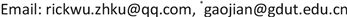3D网格模型的分析越来越成为近年来计算机图形学研究的热点问题。文中针对3D网格模型分析领域内的网格去噪平滑、网格参数化以及非刚性配准等应用问题,评述和回顾近年来应用离散微分几何理论进行分析、数学建模和算法设计的文献，介绍离散微分几何在3D模型分析领域的研究进展，并对相关问题难点和未来可能的研究方向进行了归纳和总结。 The analysis of 3D models has become a hot topic in computer graphics research in recent years. Aiming at the application problems of mesh denoising and smoothing, mesh parameterization and non-rigid registration in the field of discrete differential geometry, this paper reviews the literature on the application of discrete differential geometry theory in analysis, mathematical modeling and algorithm design in recent years, and introduces the research progress of discrete differential geometry in the field of 3D model analysis. The difficulties and possible research directions in the future are summarized.

3D网格模型分析，离散微分几何，网格光滑，网格参数化，非刚性配准, 3D Model Analysis Discrete Differential Geometry Mesh Smoothness Mesh Parameterization Non-Rigid Registration

1精密电子制造技术与装备省部共建国家重点实验室广东工业大学机电工程学院，广东 广州

2仲恺农业工程学院计算科学学院，广东 广州

3广东工贸职业技术学院机电学院，广东 广州3D网格模型的分析越来越成为近年来计算机图形学研究的热点问题。文中针对3D网格模型分析领域内的网格去噪平滑、网格参数化以及非刚性配准等应用问题,评述和回顾近年来应用离散微分几何理论进行分析、数学建模和算法设计的文献，介绍离散微分几何在3D模型分析领域的研究进展，并对相关问题难点和未来可能的研究方向进行了归纳和总结。1. 引言

2. 离散微分几何的理论框架

2.1. 基本概念

2.2. 算子

∇ u ( v ) = ∑ i u i ∇ δ i ( v ) . (1)

∇ ⋅ w ( v i ) : = ∑ f j ∈ N f ( v i ) A f j w ( f j ) ⋅ ∇ δ i | f j , (2)

Δ u = ∇ ⋅ ∇ u = ∑ j ∈ i ∗ ω i j ( u i − u j ) , (3)

( Δ − ∂ ∂ t ) u ( x , t ) = 0. (4)

h t ( x , y ) = ∑ i = 0 ∞ e − λ i t ϕ i ( x ) ϕ i ( y ) , (5)

3. 基于离散微分几何的3D模型分析

3.1. 网格去噪光滑

Δ u = − K u , (6)

u N = h ( K ) N u . (7)

3.2. 网格参数化

(1) Dirichlet能量方法的网格参数化

E ( v i ) = λ E A ( v i ) + μ E χ ( v i ) , (8)

E A ≥ 1 2 ∫ M f u × f v d u d v .

E A ( x i ) = ∑ j ∈ i * cot α i j ‖ v i − v j ‖ 2 . (9)

E χ 为欧拉示性数的变形能量，定义为：

E χ ( x i ) = ∑ j ∈ i * cot ξ i j + cot ζ i j ‖ v i − v j ‖ 2 ‖ p i − p j ‖ 2 , (10)

∂ E A ∂ p i = ∑ j ∈ i * ( cot α i j + cot β i j ) | p i − p j | = 0 (11)

∂ E χ ∂ p i = ∑ j ∈ i * ( cot ξ i j + cot ζ i j ) | p i − p j | ‖ v i − v j ‖ 2 = 0 (12)

(2) Circle packing的网格参数化

Thurston首先提出了用一系列变化的圆来近似从平面到单位圆盘的黎曼映射，从而实现网格到平面的保角映射  。文献  提出一种新的构造任意拓扑面网格到平面的离散共形映射的方法。该方法是基于圆模式(Circle packing)，即每个三角面与一个圆相对应，按照预设的夹角来调整圆的半径来达到保角映射的目的。该方法相对于调和映射有两方面的优势：1) 支持自然边界条件和规定的曲率控制边界形状的边界；2) 该解是基于作为函数的凸能量，用简单的显式表达式表示对数半径变量梯度和Hessian矩阵，因而更为稳健和高效。

d γ i d t = ( K ˜ v i − K v i ) γ i , (13)

F ( u ) = − ∫ 0 u ∑ i ( K ˜ v i − K v i ) d u i . (14)

3.3. 非刚性配准

3D刚性配准问题是指同一个实体在不同坐标系下采集得到的两个3D模型，经过刚体变换到同一个坐标系的同样姿态。这类算法是目标识别，误差估计算法的上游算法，具有广泛的应用意义，实现的算法也非常多。一般的分类如图2：

( D * , γ * ) = arg min D ∈ S O ( 3 ) , γ ∈ Γ d ˜ ( S 1 , D S 2 ∘ γ ) , (15)

L ( Θ ) = ∫ 0 1 〈 ∇ τ Θ ( τ ) , ∇ τ Θ ( τ ) 〉 1 / 2 d τ . (16)

Θ * = arg min ( D , γ ) ∈ S O ( 3 ) × Γ { min Θ : [ 0 , 1 ] → S , Θ ( 0 ) = S 1 , Θ ( 1 ) = D ( S 1 ∘ γ ) L ( Θ ) } . (17)

4. 总结